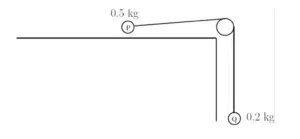# Newton’s Third Law of Motion

Newton’s Third Law of Motion says that if two bodies are in contact with one another then the force exerted on body B by body A is equal in magnitude and opposite in direction to the force exerted on body A by body B.

Newton’s Third Law of Motion – ACTION and REACTION are equal and opposite.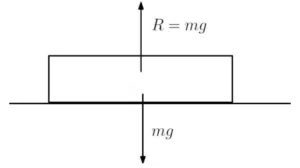You can see Newton’s Third Law of Motion in action by observing an object resting on the ground (Note that the upward force is that of the ground pushing upward on the object and the downward force is that of the object pushing downward on the ground).

As you can see, the downward force exerted on the ground by the object is the object’s mass m multiplied by gravity. It follows that, since the object doesn’t move, the ground exerts an upward reactive force on the object equal in magnitude.

Note that the same can also be said for accelerating objects. By way of example, consider a vehicle that is accelerating and pulling along a trailer.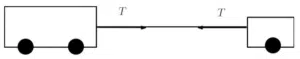The trailer has the same acceleration as the vehicle and is pulled forwards by the tension in the tow bar. Conversely, resistance from the trailer exerts an equal and opposite force on the vehicle.

If multiple objects are connected and are acting in the same straight line, they can be treated as a single object.

Click here to revise Newton’s First Law and Newton’s Second Law of Motion.

## Newton’s Third Law in Connected Particles

In Examples 1 and 2 objects in contact with one another are treated as connected particles. In addition, we assume that an object is a particle with the same weight acting at a single point. Examples 3 and 4 involve a light inextensible string over a smooth pulley. This means that we assume that the string has negligible weight, it doesn’t stretch or have friction with the pulley.

See the Mechanics area of the Edexcel A-level specification – sections 6-9, pages 34-37 of this document.

## Newton's Third Law Examples

A man of mass 80kg is using a lift that accelerates downwards at a rate of 1.5 m/s/s. Find the reactive force that the lift exerts on the man.

A 1kg scale pan carrying a 3kg block is attached to a light inextensible rope and accelerating vertically upwards at a rate of 1m/s/s. Find the tension in the rope and the force exerted on the pan by the block.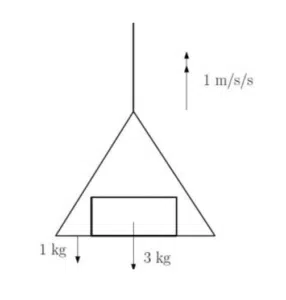Two particles of mass 3kg and 4kg are attached to the ends of a light inextensible string passing over a smooth pulley. The particles are released from rest. Find the acceleration of the particles and the tension in the string. Find the force exerted on the pulley by the string.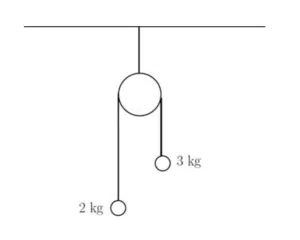Two particles P and Q are connected by a light inextensible string. Firstly, particle P has mass 0.5kg and lies on a smooth horizontal table. Secondly, particle Q has mass 0.2 kg and hangs freely over the edge of the table 80 cm from the floor. The system is released from rest. Find the time it takes for particle Q to hit the floor given that tension remains constant.